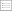Print this page

DISCUSSION PAPER PI-0820

Optimal pension asset allocation strategy when terminal utility is a function of
replacement ratio

Qing-Ping Ma

This paper considers the optimal asset allocation problem for defined-contribution
pension plan members whose terminal utility is a function of replacement ratio, i.e.
the pension-to-final wage ratio. When three asset types are available for investment,
the optimal portfolio composition, which is horizon dependent, includes investment in
both riskless and risky assets. The investment in risky assets has three components
to hedge wage risk, to speculate on risk premiums and to hedge for financial market
risk respectively.
When the terminal utility is a power function, closed form solution is derived for
the cases where there is no further contribution from wage incomes or there is no
nonhedgeable wage risk. The horizon dependence of optimal pension portfolio is
deterministic under assumptions of constant equity risk premium, constant interest rate
volatility and constant stock return volatility. The short-sale of wage replicating portfolio
also contributes to the horizon dependence of pension plan financial wealth (the sum of
pension portfolio and the short-sold wage replicating portfolio), and the effect is
stochastic due to the stochastic interest rate and stock return. Therefore, the optimal
asset allocation strategy in terms of financial wealth is “stochastic lifestyling”.
For the cases where wage incomes cannot be hedged due to non- hedgeable wage
risk, optimal asset proportions can be solved numerically by Monte Carlo simulation. The
optimal asset allocation is still horizon dependent. The proportions invested in bonds and
stocks are higher than those when wage replicating portfolio is used, hence more
shortsale of cash assets. The differences get smaller as retirement approaches.

Keywords : Optimal asset allocation; Defined-contribution pension plan; Annuity; Power
utility; Hamilton-Jacobi-Bellman equation.